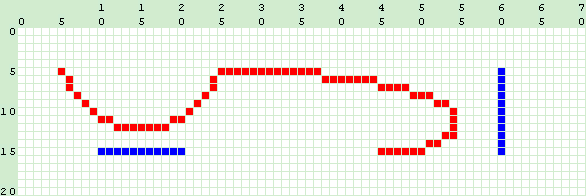Home  |  Delphi .net Home  |  System.Drawing.Graphics  |  DrawBeziers Method

DrawBeziers
Method
Draws a set of bezier curves on the current graphics canvas
 Graphics Class System.Drawing NameSpace
 NotCF 1 Procedure DrawBeziers ( Pen : System.Drawing.Pen; Points : Array of Point ) ; NotCF 2 Procedure DrawBeziers ( Pen : System.Drawing.Pen; Points : Array of PointF ) ;
CF : Methods with this mark are Compact Framework Compatible
Description
A Bezier curve is fundamental to representation of curves in graphical applications. The curve start and end points are defined, along with the same for a controlling line. A smooth curve is then drawn from the curve start point to the end, and moving close to, and parallel to the line at its centre point.

The DrawBeziers method draws a set of connected beziers - the end point of the first curve being the start point of the next and so on. So the number of points in the array should be 4 + (n*3) where the number of curves required is n-1.

The curve starts along the line between the curve and line start points. Likewise, the curve ends on the line between the curve and line end points.

The Pen object determines the style of line, its endcaps, its width and colour. By default, specifying a new pen just by colour will create a 1 pixel width line style. Endcaps allowed are defined by the System.Drawing.Drawing2D.LineCap enumeration :

 AnchorMask ArrowAnchor Custom DiamondAnchor Flat NoAnchor Round RoundAnchor Square SquareAnchor Triangle
Notes
The implementation that Microsoft have adopted appears to differ from that which is familiar in graphic art packages. Namely that the curve does not actually touch the controlling line at its centre. The MSDN documentation does not describe the algorithm used.
 Draw a pair of connected bezier curves, showing the controlling lines procedure TWinForm.TWinForm_Paint(sender: System.Object;                                   e: System.Windows.Forms.PaintEventArgs); var   myPen  : System.Drawing.Pen;   points : Array[1..7] of System.Drawing.Point; begin  // Create the pen object   myPen := System.Drawing.Pen.Create(Color.Red, 1);   // Establish the 7 points to create two bezier curves :  // 1. 1st Curve start  // 2. 1st Controlling tangential line start  // 3. 1st Controlling tangential line end  // 4. 1st Curve end  // 5. 2nd Controlling tangential line start  // 6. 2nd Controlling tangential line end  // 7. 2nd Curve end   points := Point.Create( 5,  5);   points := Point.Create(10, 15);   points := Point.Create(20, 15);   points := Point.Create(25,  5);   points := Point.Create(60,  5);   points := Point.Create(60, 15);   points := Point.Create(45, 15);   // A 1 pixel wide red bezier   e.Graphics.DrawBeziers(myPen, points);   // Now draw the controlling lines   myPen.Color := Color.blue;   e.Graphics.DrawLine(myPen, points, points);   e.Graphics.DrawLine(myPen, points, points); end; Show full unit code